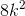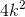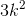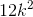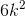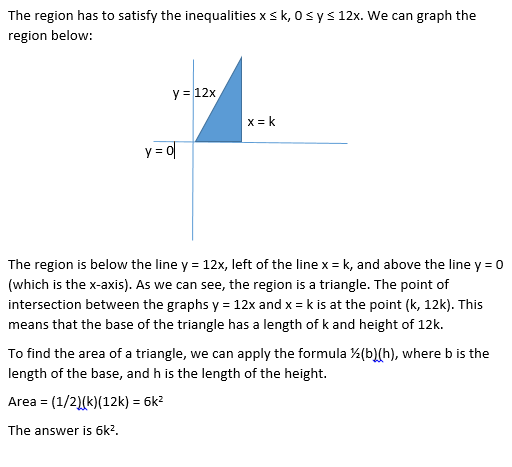## Example Questions

### Example Question #1 : How To Graph A Two Step Inequality

Solve and graph the following inequality: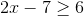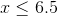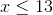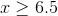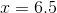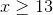Explanation:

To solve the inequality, the first step is to addto both sides: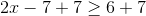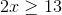The second step is to divide both sides by: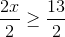To graph the inequality, you draw a straight number line. Fill in the numbers fromto infinity. Infinity can be designated by a ray. Be sure to fill in the number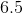, since the equation indicated greater than OR equal to.

The graph should look like:### Example Question #2 : How To Graph A Two Step Inequality

Points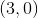and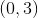lie on a circle. Which of the following could be the equation of that circle?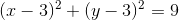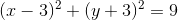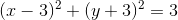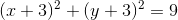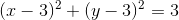Explanation:

If we plug the pointsandinto each equation, we find that these points work only in the equation. This circle has a radius ofand is centered at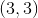.

### Example Question #3 : How To Graph A Two Step Inequality

Which of the following lines is perpendicular to the line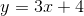?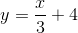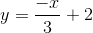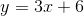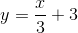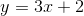Explanation:

The key here is to look for the line whose slope is the negative reciprocal of the original slope.

In this case,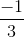is the negative reciprocal of.

Therefore, the equation of the line which is perpendicular to the original equation is:### Example Question #1 : How To Graph A Two Step Inequality

Let D be the region on the (x,y) coordinate plane that contains the solutions to the following inequalities: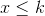, whereis a positive constant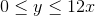Which of the following expressions, in terms of, is equivalent to the area of D?